# Electronic Circuit Simulation - Electromagnetism Background (part 1) [Python]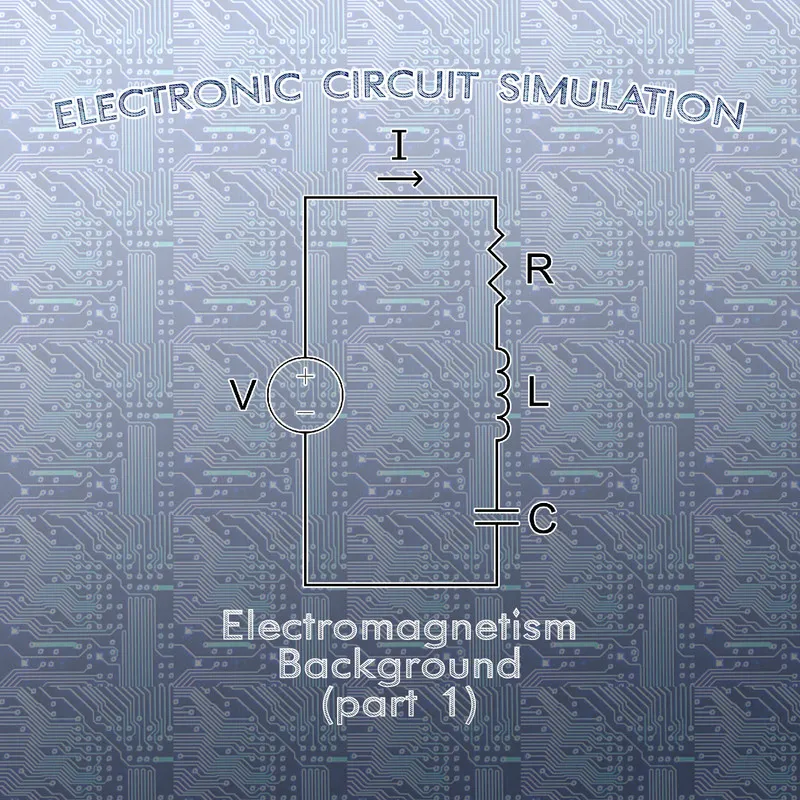[Custom Thumbnail]

All the Code of the series can be found at the Github repository:
https://github.com/drifter1/circuitsim

# Introduction

Hello it's a me again @drifter1! Today we continue with the Electric Circuit Simulation series, a tutorial series where we will be implementing a full-on electronic circuit simulator (like SPICE) studying the whole concept and mainly physics behind it! This article we will cover the following Physics Quantities:
• Electric Current
• Electric Voltage
• Electric Resistance and Conductance
• Ohm's Law
• Electric Power and Wattage

## Requirements:

• Physics and more specifically Electromagnetism Knowledge
• Knowing how to solve Linear Systems using Linear Algebra
• Some understanding of the Programming Language Python

## Difficulty:

Talking about the series in general this series can be rated:
Today's topic(s) can be rated:
• Basic

# Actual Tutorial Content

## Electric Current

Current is a physical quantity that tells us at which rate electric charge flows past a point in a circuit. Of course the electric circuit must be closed so that the charge can flow through it. On the other hand, it is also important that an Energy Supply gives potential difference (or voltage) across the two ends of the circuit. The physical quantity of electric charge, which is a physical property of matter, causing some matter to have positive (+) and other negative (-) charge, is denoted by the letter Q. Supposing that Q is the charge passing through a cross-section of a wire in time t, the electric current, symbolized by the letter I, is simply the ratio: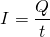In the S.I. system, Electric Current is calculated in Ampere's, often shortened to Amp or even the letter A. Electric charge is calculated in Coulomb or C. So, 1 ampere means 1 coulomb of charge passing through a cross-section of a wire every 1 second 1A = 1C / 1s.

Current in a circuit is conventionally supposed to move out of the positive pole of the battery into and into the negative pole, which is the so called conventional current direction. Thinking about the forces of opposition and attraction between electric charge (opposite charge attract, whilst same repel), we understand that this is the direction at which positive charge would move. But, as we know, in electric circuits it's electrons that actually move and so the actual natural direction is the exact opposite. Either way, it's the convention that is used everywhere and so we will still have to use the "wrong" one.

The current through specific parts of a circuit is one of the quantities that we will have to calculate in our simulator, but of course not using the ratio equation mentioned previously, as we will not dive into the "charges-level".

## Electric Voltage

One very important quantity is Electric Voltage. We previously mentioned that electric charges apply forces to each other. Those electric forces can be calculated using Coulomb's law. So, an electric charge creates some kind of field, an electric field around it, where it applies forces on charges around it, depending on it's charge and the distance of the other charge to it. As this force "acts through a distance", moving the other charge to another position, the force does some work. More specifically, bringing a charge q from a point A to a point B, near a point charge Q, we do the following Work: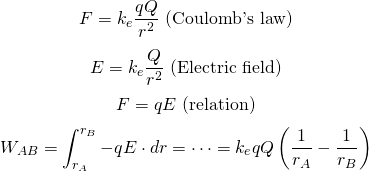The change in potential energy is equal to the work done bringing q from A to B and so the electric potential energy is defined by: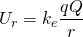In the end, the change in potential energy is: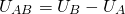Here I want you to bare with me, cause it will get a little bit confusing. What we did now is calculating the change in electric potential energy or the electric potential energy difference. To simplify our calculations more, we define something called electric potential and the so called electric potential difference, which is also known by the name Voltage!

Electric potential is given by: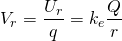So, Voltage or Electric Potential Difference is: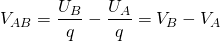Voltage is measured in volts (V). This is the second and last quantity that we will have to calculate in our simulator! Knowing both the current and voltage of each point in an electric circuit, somebody can calculate anything.

## Electric Resistance and Conductance

Different materials act differently on electric current flowing through them. The resistance to the flow of electric current and "how strongly" an material opposes this flow is defined as Electric Resistance. In the same way, the measure of "how easy" current can pass through a conductor is defined as Electric Conductance. The first is mostly symbolized by R and measured by the unit known as Ohm (Ω), whilst the second one is symbolized by G and calculated in Siemens (S). Of course the resistance and conductance are the exact inverse and so: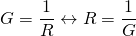Each material has some special electrical resistivity ρ and conductivity σ. Together with it's resistivity (or conductivity), the Resistance of a material is also affected by the total length (L) of the conductor, the cross-sectional area (A) of the conductor and the temperature of the conductor. In the end, the Resistance is given by:In our electric circuit simulator, the resistance of the various resistors will be known and so the value of resistance of conductance will only be used to guide us through the calculation of the various current and voltage values!

## Ohm's Law

Maybe one of the most important laws of Electromagnetism is Ohm's Law! Ohm's Law gives us the relationship between Voltage, Current and Resistance, forming the basic Relationship in form of Ohm's Law Triangle: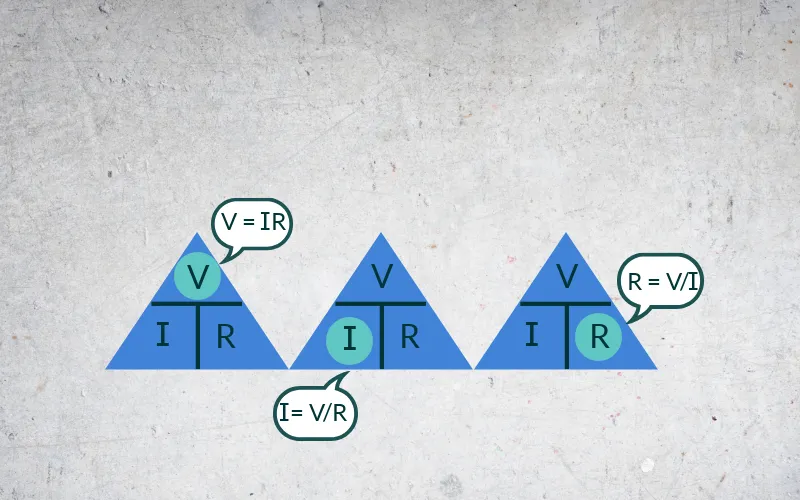[Image 1]

We will use this law a lot, cause knowing the resistance and one of the other (voltage or current), somebody can calculate the missing quantity!

## Electric Power and Wattage

Power is symbolized by the letter P and equal to the product of voltage times current. It's S.I. measurement unit is the Watt (W). Thinking about Ohm's Law we end up with the following equations for Power: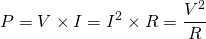Electrical Energy or Wattage is defined as the product of Power with time and measured with the common unit of energy: Joule (J). So, Wattage is given by: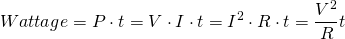Knowing the basic three quantities of Current, Voltage and Resistance for each point of an electric circuit, somebody can easily calculate the electric power of the circuit. In the same way, we could also use the electric power to calculate some of the other missing quantities!

## RESOURCES

### References:

1. https://www.physicsclassroom.com/class/circuits/Lesson-2/Electric-Current
3. https://www.electronics-tutorials.ws/resistor/resistivity.html
4. https://www.engineeringtoolbox.com/resistance-conductance-d_1654.html
5. https://www.electronics-tutorials.ws/dccircuits/dcp_2.html
6. https://owlcation.com/stem/Watt-are-Amps-and-Volts

### Images:

1.Mathematical Equations were made using quicklatex

## Final words | Next up on the project

And this is actually it for today's post! I hope that I helped you refresh your knowledge about those quantities!

Next up on this series are some more articles about the Electromagnetism Background.

So, see ya next time!

## GitHub Account:

https://github.com/drifter1Keep on drifting! ;)

H2
H3
H4
3 columns
2 columns
1 column